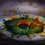# Good use of induction!!!

This is related to the Previous note about Induction

Out of the problems stated in the note, $(a)$ to $(d)$ and $(f)$ are standard results, you can use them like identities.

Here's the proof of $(e)$ which is slightly different than other ones.

Problem is to prove that $24 \mid (2\cdot 7^n+3\cdot 5^n -5)$ ...... $\forall n\in \mathbb{N}$

Observe that for $n=1$, we have $2\cdot 7^n+3\cdot 5^n -5 = 14+15-5=24$. Hence the result is true for $1$.

Assume that the result is $\color{#3D99F6}{TRUE}$ for $n=k$, and we'll prove for $n=k+1$

For this, we use a $\color{#20A900}{\text{little manipulation}}$.

Consider the term $2\cdot 7^{k+1} +3\cdot 5^{k+1} -5$.

This term is equivalent to $7(2\cdot7^k +3\cdot 5^k -5) - 6\cdot 5^k +30$ ...... this is because $7\times 3 \cdot 5^k - 6\cdot 5^k = (21-6)5^k = 3\cdot 5^{k+1}$ and this term we want in our proof!!!

Returning to the proof, we have assumed that the result is true for $n=k$, hence in the term, i.e.

$\displaystyle 2\cdot 7^{k+1} +3\cdot 5^{k+1} -5 =7 \underbrace{(2\cdot 7^k +3\cdot 5^k -5)}_{\text{divisible by 24 by assumption}} - 6\cdot 5^k +30$

Thus what only remains to prove is $-6\cdot 5^k +30$ is divisible by 24, see that

$-6\cdot 5^k+30= -6(5^k-5)$. So the problem has reduced to proving $24\mid -6(5^k -5)$ or even simpler, $4 \mid 5^k-5$

We know that $5 \equiv 1\pmod{4}\implies 5^k \equiv 1 \pmod{4}$

And $5^k\equiv 5\equiv 1 \pmod{4} \implies 5^k-5\equiv 1-1\equiv 0 \pmod{4}$

Hence $4 | 5^k-5$ . Hence the other term in the expression is also divisible by 24, thus if the result is $assumed$ to be true for $n=k$ then we are $obtaining$ it to be true for $n=k+1$ and for $n=1$ we already proved, thus it's true for $ALL$ natural numbers.Note by Aditya Raut
6 years, 10 months ago

This discussion board is a place to discuss our Daily Challenges and the math and science related to those challenges. Explanations are more than just a solution — they should explain the steps and thinking strategies that you used to obtain the solution. Comments should further the discussion of math and science.

When posting on Brilliant:

• Use the emojis to react to an explanation, whether you're congratulating a job well done , or just really confused .
• Ask specific questions about the challenge or the steps in somebody's explanation. Well-posed questions can add a lot to the discussion, but posting "I don't understand!" doesn't help anyone.
• Try to contribute something new to the discussion, whether it is an extension, generalization or other idea related to the challenge.
• Stay on topic — we're all here to learn more about math and science, not to hear about your favorite get-rich-quick scheme or current world events.

MarkdownAppears as
*italics* or _italics_ italics
**bold** or __bold__ bold
- bulleted- list
• bulleted
• list
1. numbered2. list
1. numbered
2. list
Note: you must add a full line of space before and after lists for them to show up correctly
paragraph 1paragraph 2

paragraph 1

paragraph 2

[example link](https://brilliant.org)example link
> This is a quote
This is a quote
    # I indented these lines
# 4 spaces, and now they show
# up as a code block.

print "hello world"
# I indented these lines
# 4 spaces, and now they show
# up as a code block.

print "hello world"
MathAppears as
Remember to wrap math in $$ ... $$ or $ ... $ to ensure proper formatting.
2 \times 3 $2 \times 3$
2^{34} $2^{34}$
a_{i-1} $a_{i-1}$
\frac{2}{3} $\frac{2}{3}$
\sqrt{2} $\sqrt{2}$
\sum_{i=1}^3 $\sum_{i=1}^3$
\sin \theta $\sin \theta$
\boxed{123} $\boxed{123}$

## Comments

Sort by:

Top Newest

Nice.

- 6 years, 10 months ago

Log in to reply

Good one

- 6 years, 9 months ago

Log in to reply

×

Problem Loading...

Note Loading...

Set Loading...